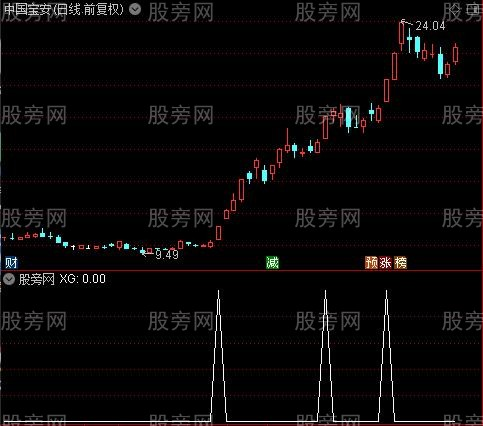# 通达信涨停起爆选股指标公式

LC:=REF(CLOSE,1);
RSI1:=SMA(MAX(CLOSE-LC,0),2,1)/SMA(ABS(CLOSE-LC),2,1)*100,COLORRED,LINETHICK2;
RSI1C:=IF(CROSS(RSI1,3) OR CROSS(RSI1,6) OR CROSS(RSI1,15) OR CROSS(RSI1,20) OR CROSS(RSI1,25) OR CROSS(RSI1,28) OR CROSS(RSI1,32) OR CROSS(RSI1,35) OR CROSS(RSI1,40) OR CROSS(RSI1,45) OR CROSS(RSI1,50) OR CROSS(RSI1,55) OR CROSS(RSI1,60) OR CROSS(RSI1,65),85,0);
RSI1Z:=IF(REF(RSI1,0)-REF(RSI1,1)>5 AND RSI1>20,65,0);
WR1:=100*(HHV(HIGH,10)-CLOSE)/(HHV(HIGH,10)-LLV(LOW,10));
WR2:=100*(HHV(HIGH,6)-CLOSE)/(HHV(HIGH,6)-LLV(LOW,6))COLORGREEN;
XG:RSI1>95.5 AND RSI1Z=65 AND WR2=0;